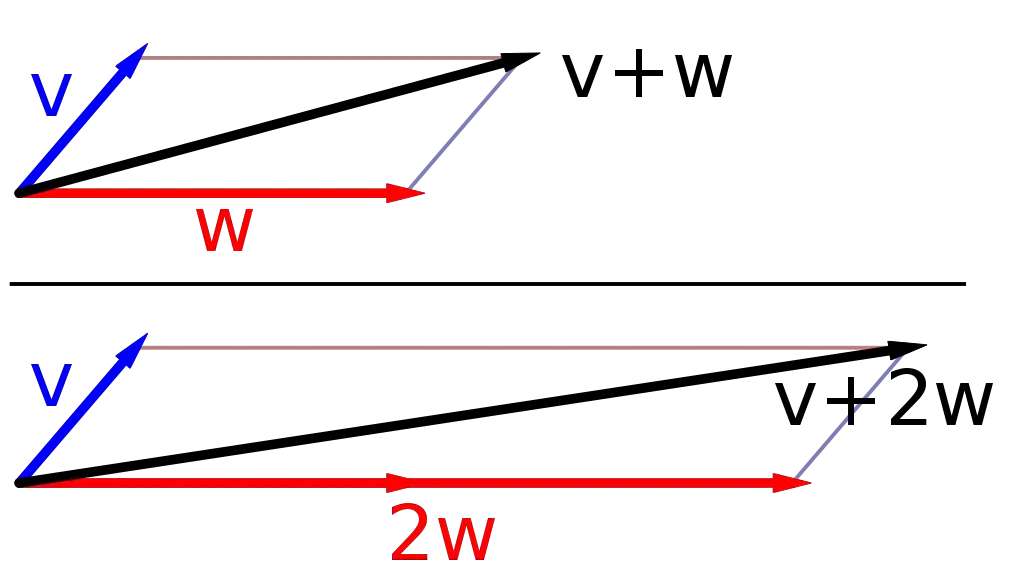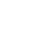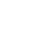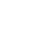# Vectors

Physics is the science that studies motion and matter and how both work with forces and energy. It is a really big subject that has a lot of different “branches” of scientific study that include motion, sound, waves, electricity, light, sound, and even astronomy.

This science covers the tiniest particles and atoms all the way to the largest objects in the universe.

Vectors are defined as a property that has both direction and magnitude. Magnitude can be thought of as a length. If you draw an arrow on a piece of paper, the magnitude’s “tail” would be the starting point and the arrow top is the “head.”

Vectors aren’t objects that you can hold or really anything that does something entertaining. Instead, vectors are a numerical value of a specific direction that is used in engineering, physics, and mathematics.

To have a vector you need both the value or “size”, and the direction. The size is called magnitude. The opposite of a vector is something called a “scalar.” They don’t have any direction, but they do have a value. Examples of scalars can include mass, temperature and energy.

In physics, vectors are drawn as arrows that have a “tail” and a “head.” The direction that the arrow is pointing is the direction of the vector and the magnitude of the arrow is the length.

### Vectors in Life

You might wonder why vectors would be important to anyone other than mathematicians, engineers or physicists. There are situations in our real world where vectors are very important to all of us.

Imagine if you were in a car and you needed to go somewhere. There aren’t any signs or streets to look at as a reference. You would need to try to figure out how to go using a map and you know where you are starting and where you will end, but not how to get there. This is where vectors are useful.

You can draw a vector between the start and finish and get on your way. As you drive along you might hit road construction and have to take detours.

Each time you do you can go back to your map and plot a new vector that will make sure you arrive where you are supposed to be. Ship captains use vectors to plot their courses on the sea because they know the direction and the speed.### Vectors can be Combined

Combining vectors is just using math. Vectors can be added together as all positive numbers or added using positive and negative numbers. Scientists use vectors as graphic representations and will sometimes have a lot of vectors working at the same time, putting them end to end.

Once they figure out the math they create a starting point and an ending point that is called the “resultant vector.”  Vectors can be represented in a lot of different ways and adding them up can give you different results, depending upon each vector direction.

Vectors can exist in shapes that involve everything from a simple single arrow to complex shapes and sizes. When you have a lot of complexity in shape, the instructions for adding gets really confusing.

One way is to use the head-to-tail method which is just starting at the tail of one arrow and following it to its head and taking that number first. Then follow the tail that is touching the previous arrow’s head to the next, and so on; adding each number as you go.

Vectors can be defined in both two and three dimensional space.

• Vector graphics are used in computers because they can be enlarged without losing the quality of the image.
• A unit vector has a magnitude of “1” and is used to define direction.
• Some of the forces that are added to direction that are vector quantities include thrust, weight moving forward, speed, acceleration, and velocity.

### Q&A:

What is a vector?
a property that has both direction and magnitude

What is a scalar?
a property that has value but no direction

Are all vectors the same?
no

Name one area where vectors are used.
engineering, physics, mathematics

Are all vectors in the shape of a triangle?
no

What are the two parts of the vector arrow?
•History
•Science
•Geography
•Biography
•US History